# 6.2 Graphs of exponential functions  (Page 6/6)

 Page 6 / 6

## Verbal

What role does the horizontal asymptote of an exponential function play in telling us about the end behavior of the graph?

An asymptote is a line that the graph of a function approaches, as $\text{\hspace{0.17em}}x\text{\hspace{0.17em}}$ either increases or decreases without bound. The horizontal asymptote of an exponential function tells us the limit of the function’s values as the independent variable gets either extremely large or extremely small.

What is the advantage of knowing how to recognize transformations of the graph of a parent function algebraically?

## Algebraic

The graph of $\text{\hspace{0.17em}}f\left(x\right)={3}^{x}\text{\hspace{0.17em}}$ is reflected about the y -axis and stretched vertically by a factor of $\text{\hspace{0.17em}}4.\text{\hspace{0.17em}}$ What is the equation of the new function, $\text{\hspace{0.17em}}g\left(x\right)?\text{\hspace{0.17em}}$ State its y -intercept, domain, and range.

$g\left(x\right)=4{\left(3\right)}^{-x};\text{\hspace{0.17em}}$ y -intercept: $\text{\hspace{0.17em}}\left(0,4\right);\text{\hspace{0.17em}}$ Domain: all real numbers; Range: all real numbers greater than $\text{\hspace{0.17em}}0.$

The graph of $\text{\hspace{0.17em}}f\left(x\right)={\left(\frac{1}{2}\right)}^{-x}\text{\hspace{0.17em}}$ is reflected about the y -axis and compressed vertically by a factor of $\text{\hspace{0.17em}}\frac{1}{5}.\text{\hspace{0.17em}}$ What is the equation of the new function, $\text{\hspace{0.17em}}g\left(x\right)?\text{\hspace{0.17em}}$ State its y -intercept, domain, and range.

The graph of $\text{\hspace{0.17em}}f\left(x\right)={10}^{x}\text{\hspace{0.17em}}$ is reflected about the x -axis and shifted upward $\text{\hspace{0.17em}}7\text{\hspace{0.17em}}$ units. What is the equation of the new function, $\text{\hspace{0.17em}}g\left(x\right)?\text{\hspace{0.17em}}$ State its y -intercept, domain, and range.

$g\left(x\right)=-{10}^{x}+7;\text{\hspace{0.17em}}$ y -intercept: $\text{\hspace{0.17em}}\left(0,6\right);\text{\hspace{0.17em}}$ Domain: all real numbers; Range: all real numbers less than $\text{\hspace{0.17em}}7.$

The graph of $\text{\hspace{0.17em}}f\left(x\right)={\left(1.68\right)}^{x}\text{\hspace{0.17em}}$ is shifted right $\text{\hspace{0.17em}}3\text{\hspace{0.17em}}$ units, stretched vertically by a factor of $\text{\hspace{0.17em}}2,$ reflected about the x -axis, and then shifted downward $\text{\hspace{0.17em}}3\text{\hspace{0.17em}}$ units. What is the equation of the new function, $\text{\hspace{0.17em}}g\left(x\right)?\text{\hspace{0.17em}}$ State its y -intercept (to the nearest thousandth), domain, and range.

The graph of $\text{\hspace{0.17em}}f\left(x\right)=2{\left(\frac{1}{4}\right)}^{x-20}$ is shifted left $\text{\hspace{0.17em}}2\text{\hspace{0.17em}}$ units, stretched vertically by a factor of $\text{\hspace{0.17em}}4,$ reflected about the x -axis, and then shifted downward $\text{\hspace{0.17em}}4\text{\hspace{0.17em}}$ units. What is the equation of the new function, $\text{\hspace{0.17em}}g\left(x\right)?\text{\hspace{0.17em}}$ State its y -intercept, domain, and range.

$g\left(x\right)=2{\left(\frac{1}{4}\right)}^{x};\text{\hspace{0.17em}}$ y -intercept: Domain: all real numbers; Range: all real numbers greater than $\text{\hspace{0.17em}}0.$

## Graphical

For the following exercises, graph the function and its reflection about the y -axis on the same axes, and give the y -intercept.

$f\left(x\right)=3{\left(\frac{1}{2}\right)}^{x}$

$g\left(x\right)=-2{\left(0.25\right)}^{x}$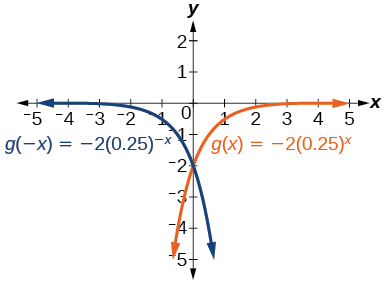y -intercept: $\text{\hspace{0.17em}}\left(0,-2\right)$

$h\left(x\right)=6{\left(1.75\right)}^{-x}$

For the following exercises, graph each set of functions on the same axes.

$f\left(x\right)=3{\left(\frac{1}{4}\right)}^{x},$ $g\left(x\right)=3{\left(2\right)}^{x},$ and $\text{\hspace{0.17em}}h\left(x\right)=3{\left(4\right)}^{x}$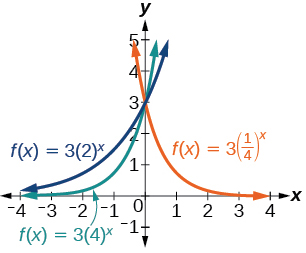$f\left(x\right)=\frac{1}{4}{\left(3\right)}^{x},$ $g\left(x\right)=2{\left(3\right)}^{x},$ and $\text{\hspace{0.17em}}h\left(x\right)=4{\left(3\right)}^{x}$

For the following exercises, match each function with one of the graphs in [link] .

$f\left(x\right)=2{\left(0.69\right)}^{x}$

B

$f\left(x\right)=2{\left(1.28\right)}^{x}$

$f\left(x\right)=2{\left(0.81\right)}^{x}$

A

$f\left(x\right)=4{\left(1.28\right)}^{x}$

$f\left(x\right)=2{\left(1.59\right)}^{x}$

E

$f\left(x\right)=4{\left(0.69\right)}^{x}$

For the following exercises, use the graphs shown in [link] . All have the form $\text{\hspace{0.17em}}f\left(x\right)=a{b}^{x}.$

Which graph has the largest value for $\text{\hspace{0.17em}}b?$

D

Which graph has the smallest value for $\text{\hspace{0.17em}}b?$

Which graph has the largest value for $\text{\hspace{0.17em}}a?$

C

Which graph has the smallest value for $\text{\hspace{0.17em}}a?$

For the following exercises, graph the function and its reflection about the x -axis on the same axes.

$f\left(x\right)=\frac{1}{2}{\left(4\right)}^{x}$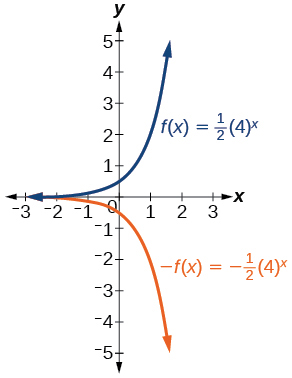$f\left(x\right)=3{\left(0.75\right)}^{x}-1$

$f\left(x\right)=-4{\left(2\right)}^{x}+2$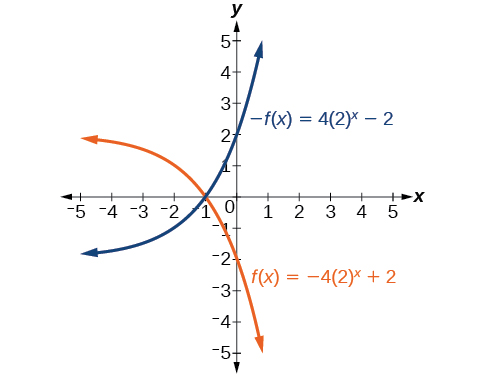For the following exercises, graph the transformation of $\text{\hspace{0.17em}}f\left(x\right)={2}^{x}.\text{\hspace{0.17em}}$ Give the horizontal asymptote, the domain, and the range.

$f\left(x\right)={2}^{-x}$

$h\left(x\right)={2}^{x}+3$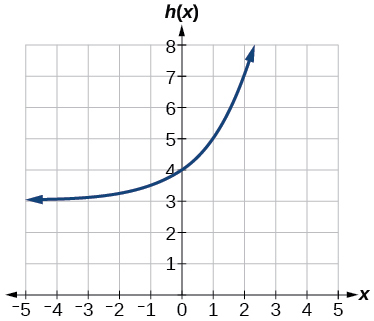Horizontal asymptote: $\text{\hspace{0.17em}}h\left(x\right)=3;$ Domain: all real numbers; Range: all real numbers strictly greater than $\text{\hspace{0.17em}}3.$

$f\left(x\right)={2}^{x-2}$

For the following exercises, describe the end behavior of the graphs of the functions.

$f\left(x\right)=-5{\left(4\right)}^{x}-1$

As $x\to \infty$ , $f\left(x\right)\to -\infty$ ;
As $x\to -\infty$ , $f\left(x\right)\to -1$

$f\left(x\right)=3{\left(\frac{1}{2}\right)}^{x}-2$

$f\left(x\right)=3{\left(4\right)}^{-x}+2$

As $x\to \infty$ , $f\left(x\right)\to 2$ ;
As $x\to -\infty$ , $f\left(x\right)\to \infty$

For the following exercises, start with the graph of $\text{\hspace{0.17em}}f\left(x\right)={4}^{x}.\text{\hspace{0.17em}}$ Then write a function that results from the given transformation.

Shift $f\left(x\right)$ 4 units upward

Shift $\text{\hspace{0.17em}}f\left(x\right)\text{\hspace{0.17em}}$ 3 units downward

$f\left(x\right)={4}^{x}-3$

Shift $\text{\hspace{0.17em}}f\left(x\right)\text{\hspace{0.17em}}$ 2 units left

Shift $\text{\hspace{0.17em}}f\left(x\right)\text{\hspace{0.17em}}$ 5 units right

$f\left(x\right)={4}^{x-5}$

Reflect $\text{\hspace{0.17em}}f\left(x\right)\text{\hspace{0.17em}}$ about the x -axis

Reflect $\text{\hspace{0.17em}}f\left(x\right)\text{\hspace{0.17em}}$ about the y -axis

$f\left(x\right)={4}^{-x}$

For the following exercises, each graph is a transformation of $\text{\hspace{0.17em}}y={2}^{x}.\text{\hspace{0.17em}}$ Write an equation describing the transformation.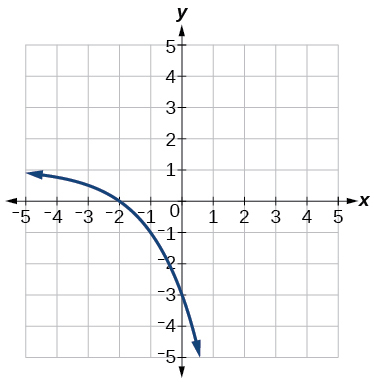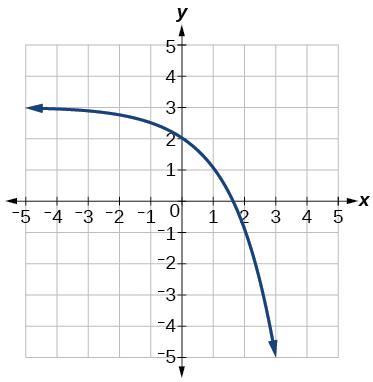$y=-{2}^{x}+3$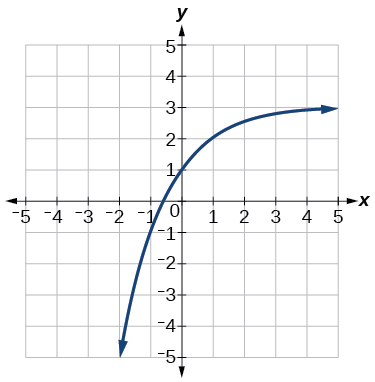For the following exercises, find an exponential equation for the graph.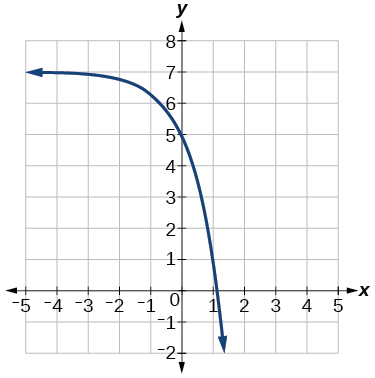$y=-2{\left(3\right)}^{x}+7$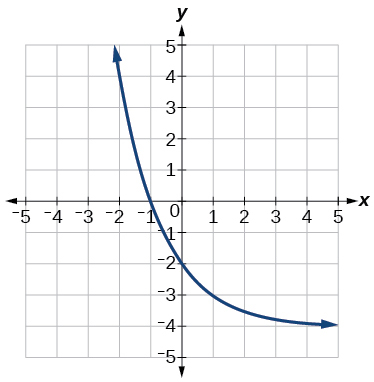## Numeric

For the following exercises, evaluate the exponential functions for the indicated value of $\text{\hspace{0.17em}}x.$

$g\left(x\right)=\frac{1}{3}{\left(7\right)}^{x-2}\text{\hspace{0.17em}}$ for $\text{\hspace{0.17em}}g\left(6\right).$

$g\left(6\right)=800+\frac{1}{3}\approx 800.3333$

$f\left(x\right)=4{\left(2\right)}^{x-1}-2\text{\hspace{0.17em}}$ for $\text{\hspace{0.17em}}f\left(5\right).$

$h\left(x\right)=-\frac{1}{2}{\left(\frac{1}{2}\right)}^{x}+6\text{\hspace{0.17em}}$ for $\text{\hspace{0.17em}}h\left(-7\right).$

$h\left(-7\right)=-58$

## Technology

For the following exercises, use a graphing calculator to approximate the solutions of the equation. Round to the nearest thousandth. $\text{\hspace{0.17em}}f\left(x\right)=a{b}^{x}+d.$

$-50=-{\left(\frac{1}{2}\right)}^{-x}$

$116=\frac{1}{4}{\left(\frac{1}{8}\right)}^{x}$

$x\approx -2.953$

$12=2{\left(3\right)}^{x}+1$

$5=3{\left(\frac{1}{2}\right)}^{x-1}-2$

$x\approx -0.222$

$-30=-4{\left(2\right)}^{x+2}+2$

## Extensions

Explore and discuss the graphs of $\text{\hspace{0.17em}}F\left(x\right)={\left(b\right)}^{x}\text{\hspace{0.17em}}$ and $\text{\hspace{0.17em}}G\left(x\right)={\left(\frac{1}{b}\right)}^{x}.\text{\hspace{0.17em}}$ Then make a conjecture about the relationship between the graphs of the functions $\text{\hspace{0.17em}}{b}^{x}\text{\hspace{0.17em}}$ and $\text{\hspace{0.17em}}{\left(\frac{1}{b}\right)}^{x}\text{\hspace{0.17em}}$ for any real number $\text{\hspace{0.17em}}b>0.$

The graph of $\text{\hspace{0.17em}}G\left(x\right)={\left(\frac{1}{b}\right)}^{x}\text{\hspace{0.17em}}$ is the refelction about the y -axis of the graph of $\text{\hspace{0.17em}}F\left(x\right)={b}^{x};\text{\hspace{0.17em}}$ For any real number $\text{\hspace{0.17em}}b>0\text{\hspace{0.17em}}$ and function $\text{\hspace{0.17em}}f\left(x\right)={b}^{x},$ the graph of $\text{\hspace{0.17em}}{\left(\frac{1}{b}\right)}^{x}\text{\hspace{0.17em}}$ is the the reflection about the y -axis, $\text{\hspace{0.17em}}F\left(-x\right).$

Prove the conjecture made in the previous exercise.

Explore and discuss the graphs of $\text{\hspace{0.17em}}f\left(x\right)={4}^{x},$ $\text{\hspace{0.17em}}g\left(x\right)={4}^{x-2},$ and $\text{\hspace{0.17em}}h\left(x\right)=\left(\frac{1}{16}\right){4}^{x}.\text{\hspace{0.17em}}$ Then make a conjecture about the relationship between the graphs of the functions $\text{\hspace{0.17em}}{b}^{x}\text{\hspace{0.17em}}$ and $\text{\hspace{0.17em}}\left(\frac{1}{{b}^{n}}\right){b}^{x}\text{\hspace{0.17em}}$ for any real number n and real number $\text{\hspace{0.17em}}b>0.$

The graphs of $\text{\hspace{0.17em}}g\left(x\right)\text{\hspace{0.17em}}$ and $\text{\hspace{0.17em}}h\left(x\right)\text{\hspace{0.17em}}$ are the same and are a horizontal shift to the right of the graph of $\text{\hspace{0.17em}}f\left(x\right);\text{\hspace{0.17em}}$ For any real number n , real number $\text{\hspace{0.17em}}b>0,$ and function $\text{\hspace{0.17em}}f\left(x\right)={b}^{x},$ the graph of $\text{\hspace{0.17em}}\left(\frac{1}{{b}^{n}}\right){b}^{x}\text{\hspace{0.17em}}$ is the horizontal shift $\text{\hspace{0.17em}}f\left(x-n\right).$

Prove the conjecture made in the previous exercise.

root under 3-root under 2 by 5 y square
The sum of the first n terms of a certain series is 2^n-1, Show that , this series is Geometric and Find the formula of the n^th
cosA\1+sinA=secA-tanA
why two x + seven is equal to nineteen.
The numbers cannot be combined with the x
Othman
2x + 7 =19
humberto
2x +7=19. 2x=19 - 7 2x=12 x=6
Yvonne
because x is 6
SAIDI
what is the best practice that will address the issue on this topic? anyone who can help me. i'm working on my action research.
simplify each radical by removing as many factors as possible (a) √75
how is infinity bidder from undefined?
what is the value of x in 4x-2+3
give the complete question
Shanky
4x=3-2 4x=1 x=1+4 x=5 5x
Olaiya
hi can you give another equation I'd like to solve it
Daniel
what is the value of x in 4x-2+3
Olaiya
if 4x-2+3 = 0 then 4x = 2-3 4x = -1 x = -(1÷4) is the answer.
Jacob
4x-2+3 4x=-3+2 4×=-1 4×/4=-1/4
LUTHO
then x=-1/4
LUTHO
4x-2+3 4x=-3+2 4x=-1 4x÷4=-1÷4 x=-1÷4
LUTHO
A research student is working with a culture of bacteria that doubles in size every twenty minutes. The initial population count was  1350  bacteria. Rounding to five significant digits, write an exponential equation representing this situation. To the nearest whole number, what is the population size after  3  hours?
v=lbh calculate the volume if i.l=5cm, b=2cm ,h=3cm
Need help with math
Peya
can you help me on this topic of Geometry if l help you
litshani
( cosec Q _ cot Q ) whole spuare = 1_cosQ / 1+cosQ
A guy wire for a suspension bridge runs from the ground diagonally to the top of the closest pylon to make a triangle. We can use the Pythagorean Theorem to find the length of guy wire needed. The square of the distance between the wire on the ground and the pylon on the ground is 90,000 feet. The square of the height of the pylon is 160,000 feet. So, the length of the guy wire can be found by evaluating √(90000+160000). What is the length of the guy wire?
the indicated sum of a sequence is known as
how do I attempted a trig number as a starter
cos 18 ____ sin 72 evaluateByByBy Szilárd JankóBy JavaChamp TeamBy Stephen VoronBy RhodesByBy Michael SagBy OpenStaxByBy Mary CohenBy Qqq Qqq